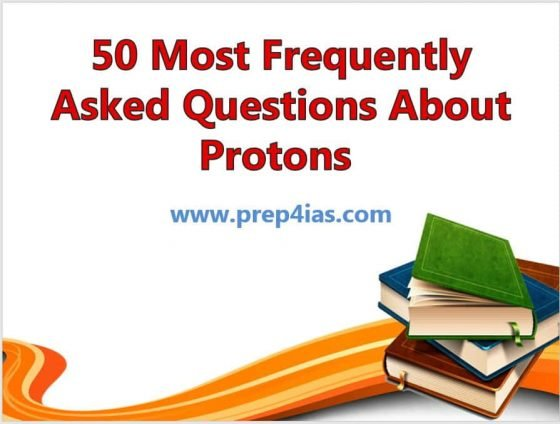In this article, I will take you through 50 Most Frequently Asked Questions About Protons that every learner must be aware of. The word proton is Greek for "first", and this name was given to the hydrogen nucleus by Ernest Rutherford in 1920. In previous years, Rutherford had discovered that the hydrogen nucleus (known to be the lightest nucleus) could be extracted from the nuclei of nitrogen by atomic collisions. Protons were therefore a candidate to be a fundamental or elementary particle, and hence a building block of nitrogen and all other heavier atomic nuclei. We will see some of the most frequently asked questions about protons in below section. More on wikipedia.### 1. What is a Proton ?

Ans. A proton is a stable subatomic particle occurring in all atomic nuclei with a positive electric charge equal in magnitude to that of an electron.

### 2. Who discovered the Proton ?

Ans. Ernest Rutherford

Ans. 6 protons

Ans. 8 protons

Ans. 3 protons

### 6. Where are protons located in an Atom ?

Ans. Protons are located in the nucleus of an Atom.

### 7. What is the charge of a Proton ?

Ans. A proton carries a positive charge equal in magnitude to a unit of electron charge.

Ans. 11 protons

### 9. How to find number of Protons in an Atom ?

Ans. The number of protons in an atom is calculated by its atomic number.

### 10. Do Isotopes have the same number of Protons ?

Ans. Isotopes are members of a family of an element that all have the same number of protons but different numbers of neutrons.

Ans. 20 protons

Ans. 1 proton

Ans. 7 protons

Ans. 19 protons

### 15. What is the mass of a Proton ?

Ans. 1.6726219 × 10^-27 kilograms

### 16. Do Protons and Electrons have the same mass ?

Ans. No, Protons are much heavier than the electrons.

Ans. 17 Protons

Ans. 15 Protons

Ans. 20 Protons

Ans. 13 Protons

Ans. 5 Proton

Ans. 29 Protons

Ans. 2 Protons

Ans. 26 Protons

Ans. 12 Protons

Ans. 10 Protons

Ans. 47 Protons

### 28. What are protons made of ?

Ans. Protons are made of fast-moving point of energy called Quarks. Each proton contains three quarks.

Ans. 8 protons

Ans. 11 protons

Ans. 9 protons

Ans. 79 protons

Ans. 14 protons

Ans. 16 Protons

Ans. 22 protons

Ans. 4 protons

### 37. Are protons smaller than electron ?

Ans. No, it is the opposite. Electrons are over 1800 times smaller than protons.

### 38. Do electrons and protons attract each other ?

Ans. Since electrons carry negative charge and protons carry positive charge and we know opposite charges attracts each other so protons and electrons attract each other.

Ans. 13 Protons

Ans. 18 Protons

Ans. 53 Protons

Ans. 82 Protons

Ans. 80 protons

Ans. 18 protons

### 45. Does Mercury have more proton and electrons than Tin ?

Ans. Yes, Mercury have more proton and electrons than Tin.

### 46. Is proton heavier than neutrons ?

Ans. No, it is neutron which is slightly heavier than proton in a nucleus.

### 47. How can Protons be transformed to Neutrons ?

Ans. Protons are known to transform into neutrons through the process of electron capture (also called inverse beta decay).

### 48. How can Neutrons be transformed back to Protons ?

Ans. Neutrons can convert back to protons through beta decay, a common form of radioactive decay.

### 49. What is the accepted value of a proton's charge radius ?

Ans. It is 0.8768 fm.

Ans. 78 protons